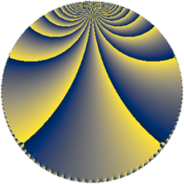# Properties

 Label 648.4.dLevel $648$ Weight $4$ Character orbit 648.d Rep. character $\chi_{648}(325,\cdot)$ Character field $\Q$ Dimension $140$ Sturm bound $432$

# Related objects

## Defining parameters

 Level: $$N$$ $$=$$ $$648 = 2^{3} \cdot 3^{4}$$ Weight: $$k$$ $$=$$ $$4$$ Character orbit: $$[\chi]$$ $$=$$ 648.d (of order $$2$$ and degree $$1$$) Character conductor: $$\operatorname{cond}(\chi)$$ $$=$$ $$8$$ Character field: $$\Q$$ Sturm bound: $$432$$

## Dimensions

The following table gives the dimensions of various subspaces of $$M_{4}(648, [\chi])$$.

Total New Old
Modular forms 336 148 188
Cusp forms 312 140 172
Eisenstein series 24 8 16

## Trace form

 $$140q + 2q^{4} + 4q^{7} + O(q^{10})$$ $$140q + 2q^{4} + 4q^{7} - 20q^{10} + 182q^{16} + 70q^{22} - 3096q^{25} + 376q^{28} + 4q^{31} + 414q^{34} - 68q^{40} + 948q^{46} + 5688q^{49} + 1176q^{52} - 508q^{55} - 1892q^{58} - 958q^{64} + 3656q^{70} - 8q^{73} + 2826q^{76} - 716q^{79} - 474q^{82} - 5522q^{88} + 276q^{94} + 4q^{97} + O(q^{100})$$

## Decomposition of $$S_{4}^{\mathrm{new}}(648, [\chi])$$ into newform subspaces

The newforms in this space have not yet been added to the LMFDB.

## Decomposition of $$S_{4}^{\mathrm{old}}(648, [\chi])$$ into lower level spaces

$$S_{4}^{\mathrm{old}}(648, [\chi]) \cong$$ $$S_{4}^{\mathrm{new}}(8, [\chi])$$$$^{\oplus 5}$$$$\oplus$$$$S_{4}^{\mathrm{new}}(24, [\chi])$$$$^{\oplus 4}$$$$\oplus$$$$S_{4}^{\mathrm{new}}(72, [\chi])$$$$^{\oplus 3}$$$$\oplus$$$$S_{4}^{\mathrm{new}}(216, [\chi])$$$$^{\oplus 2}$$